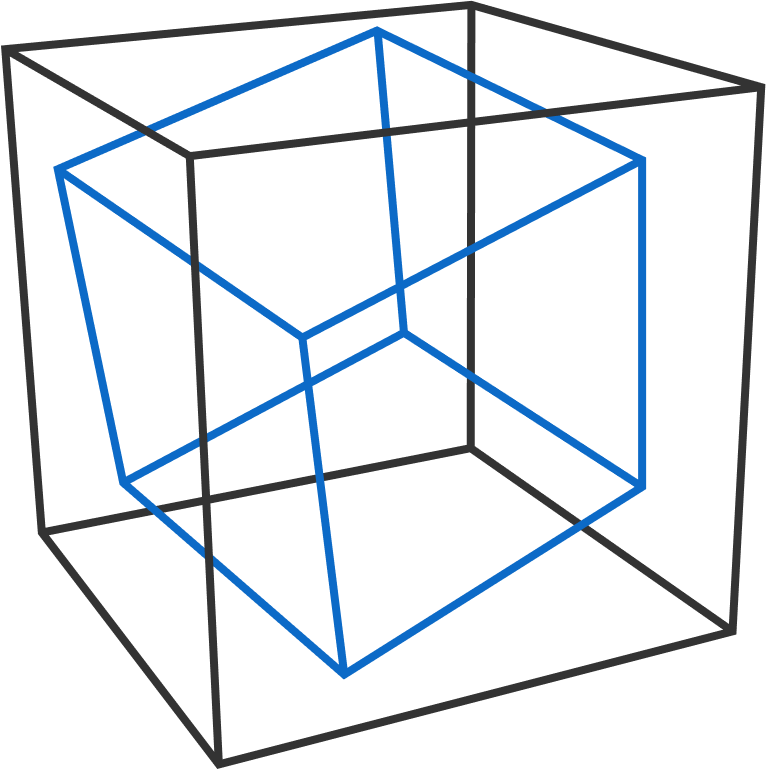# Cube Inscribed In Cube

Geometry Level 3A cube is inscribed in a unit cube so that exactly 6 of the inscribed cube's 8 vertices each touch a different face of the unit cube.

What's the smallest cube that can be inscribed in this way?

If its side length can be expressed as $\frac{a}{b},$ where $a$ and $b$ are coprime positive integers, give the product $a \times b$ as the answer.

×